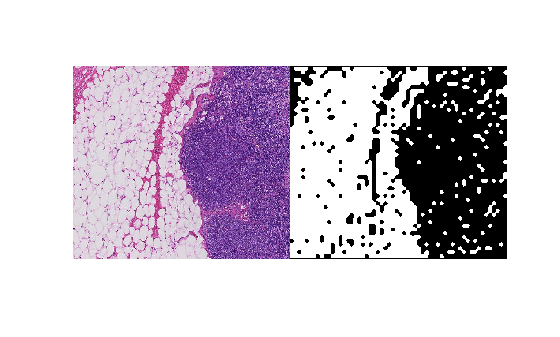# world2sub

Convert world coordinates to pixel subscripts

Since R2021a

## Syntax

``pixelsub = world2sub(bim,world)``
``pixelsub = world2sub(bim,world,Level=L)``

## Description

example

````pixelsub = world2sub(bim,world)` converts the world coordinates, `world`, to the corresponding pixel subscripts, `pixelsub`. The world coordinates should be in the same order as the pixel subscripts.```
````pixelsub = world2sub(bim,world,Level=L)` additionally specifies the resolution level `L` to use in a multiresolution image.```

## Examples

collapse all

Convert pixel subscripts from one level to another via the world coordinates.

Create a blocked image from a sample image included with the toolbox.

`bim = blockedImage("tumor_091R.tif");`

Create a binary mask at the coarsest resolution level.

`bmask = apply(bim, @(bs)imbinarize(im2gray(bs.Data)),Level=3);`

Define a region of interest (ROI) in the finest resolution level in pixel subscripts.

```pixelStartLevel1 = [1700 1550]; pixelEndLevel1 = [2100 2000]; imagePixelSubs = [pixelStartLevel1; pixelEndLevel1];```

Get the image data from the ROI at the finest resolution level.

```roiImage = getRegion(bim,pixelStartLevel1,pixelEndLevel1,Level=1); size(roiImage)```
```ans = 1×3 401 451 3 ```

Convert the pixel subscripts that define the ROI into world coordinates. By default, `sub2world` converts the coordinates at the finest resolution level.

`worldRegion = sub2world(bim,imagePixelSubs);`

Convert the world coordinates of the ROI to pixel subscripts of the mask.

`maskPixelSubs = world2sub(bmask,worldRegion);`

Get the mask data from the ROI.

```roiMask = getRegion(bmask,maskPixelSubs(1,:),maskPixelSubs(2,:)); size(roiMask)```
```ans = 1×2 51 58 ```

View the region of interest and the corresponding mask.

`montage({roiImage,roiMask})`## Input Arguments

collapse all

Blocked image, specified as a `blockedImage` object.

World coordinates, specified as a K-by-N numeric matrix, where K is the number of world coordinate vectors. N is equal to or less than the number of dimensions of the blocked image `bim`. For example, you can exclude a color channel from the conversion by specifying the world coordinates with one less dimension than the blocked image.

Data Types: `single` | `double` | `int8` | `int16` | `int32` | `int64` | `uint8` | `uint16` | `uint32` | `uint64` | `logical`

Resolution level, specified as a positive integer that is less than or equal to the number of resolution levels of `bim`.

## Output Arguments

collapse all

Pixel subscripts, returned as a K-by-N integer-valued matrix, where K is the number of coordinates and N is the dimensionality of the world coordinates.

## Tips

• World coordinates are theoretically continuous domain values represented by floating point numbers. Subscripts are discrete integer values that can be used to index into the underlying array. Floating point computation and rounding may cause small changes in world coordinates around the edge of pixels to map to different neighboring subscript locations. `world2sub` rounds up world coordinate values on the edge of two pixels, except for pixels on the border, where it rounds down to the last pixel.

## Version History

Introduced in R2021a

expand all